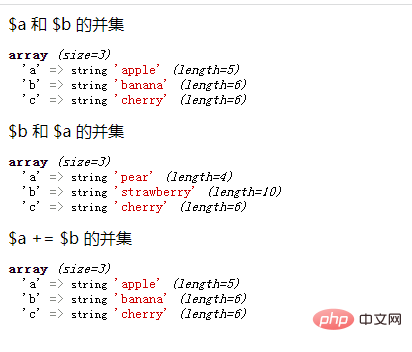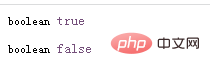# php中数组操作运算符有哪些

php中数组运算符有6个：1、“+”符，用于把右边的数组元素附加到左边的数组后面，如果存在相同键名，则左边的数组值会覆盖掉右边的数组值；2、“==”符，用于比较两个数组是否相等；3、“===”符，用于比较两个数组是否全等；4、“!=”符等。php中的数组操作运算符

+联合\$a + \$b\$a\$b的联合。
==相等\$a == \$b如果\$a\$b具有相同的键／值对则为 `true`
===全等\$a === \$b如果\$a\$b具有相同的键／值对并且顺序和类型都相同则为 `true`
!=不等\$a != \$b如果\$a不等于\$b则为 `true`
<>不等\$a <> \$b如果\$a不等于\$b则为 `true`
!==不全等\$a !== \$b如果\$a不全等于\$b则为 `true`

1、+ 运算符

+ 运算符把右边的数组元素附加到左边的数组后面，如果键相同的情况下，前面的数组值会覆盖掉后面的数组值。

```<?php
\$a = array("a" => "apple", "b" => "banana");
\$b = array("a" => "pear", "b" => "strawberry", "c" => "cherry");

\$c = \$a + \$b; // \$a 和 \$b 的并集
echo "\\$a 和 \\$b 的并集";
var_dump(\$c);

\$c = \$b + \$a; // \$b 和 \$a 的并集
echo "\\$b 和 \\$a 的并集";
var_dump(\$c);

\$a += \$b; //  \$a += \$b 的并集
echo "\\$a += \\$b 的并集";
var_dump(\$a);
?>```2、比较运算符

==（等于），===（恒等于） ，<>（不等于），!=（不等于）， !==（不全等）

```<?php
\$a = array("apple", "banana");
\$b = array(1 => "banana", "0" => "apple");

var_dump(\$a == \$b); // bool(true)
var_dump(\$a === \$b); // bool(false)
?>```1/1